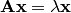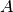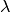Warning

This documents an unmaintained version of NetworkX. Please upgrade to a maintained version and see the current NetworkX documentation.

# eigenvector_centrality¶

eigenvector_centrality(G, max_iter=100, tol=1e-06, nstart=None, weight='weight')[source]

Compute the eigenvector centrality for the graph G.

Eigenvector centrality computes the centrality for a node based on the centrality of its neighbors. The eigenvector centrality for nodeiswhereis the adjacency matrix of the graph G with eigenvalue. By virtue of the Perron–Frobenius theorem, there is a unique and positive solution ifis the largest eigenvalue associated with the eigenvector of the adjacency matrix().

Parameters: G (graph) – A networkx graph max_iter (integer, optional) – Maximum number of iterations in power method. tol (float, optional) – Error tolerance used to check convergence in power method iteration. nstart (dictionary, optional) – Starting value of eigenvector iteration for each node. weight (None or string, optional) – If None, all edge weights are considered equal. Otherwise holds the name of the edge attribute used as weight. nodes – Dictionary of nodes with eigenvector centrality as the value. dictionary

Examples

>>> G = nx.path_graph(4)
>>> centrality = nx.eigenvector_centrality(G)
>>> print(['%s %0.2f'%(node,centrality[node]) for node in centrality])
['0 0.37', '1 0.60', '2 0.60', '3 0.37']


eigenvector_centrality_numpy(), pagerank(), hits(), Notes(), ------(), The(), The(), no(), iterations(), reached.(), For(), to(), first()